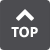##M Machine Vibration Vibrations arising from the mechanical system. Margin The percentage of contact between a cam and a cam follower. Recommended margin is at least 60. Misalignment The error in the alignment of the centers of two or more shafts coupled to each other. Misalignment can be in the form of deviation angle error, clearance error and parallelism error. Modified Constant Velocity, MCV Curve A curve with a stretch that produces a uniform speed curve. Used when the maximum speed must be reduced to suppress the pressure angle or when a stretch of equivalent speed is necessary. Modified Sine, MS Curve A standard and widely used cam curve that has a low maximum speed rating and small torque coefficient for the cam shaft. The acceleration is also relatively small making it suitable for applications in which the nature of the load is unknown. Modified Trapezoid, MT Curve A standard curve designed for minimum acceleration. Particularly suitable for lightweight loads at high speeds. Moment The rotational driving force represented by the product of the radius and the force. Moment of Inertia The product of the mass multiplied by its distance r from a given straight line raised to the power of two. I = ∫r2dm. When using within the industrial unit system, the equation GD2 = 4gI gives the relationship with GD2 where g is the gravitational acceleration. Motion Curve The motion of an object expressed by function s = S (t) of time t. Motion curves are characterized by its monovalent function and derivability.